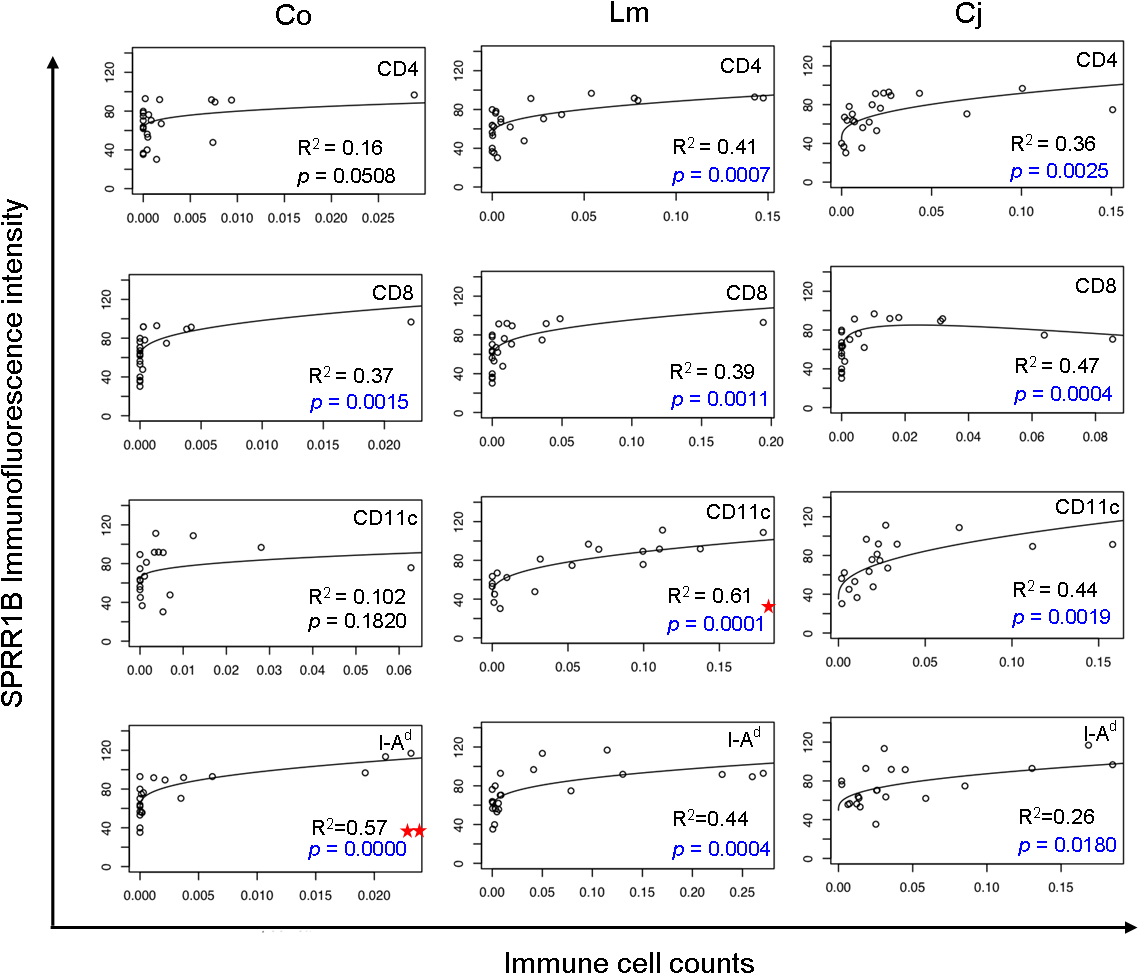Figure 7 of Chen, Mol Vis 2009; 15:563-576.

Figure 7. Immune cell infiltration of the ocular surface predicts corneal SPRR1B expression. For each of the 12 subfigures, we plotted SPRR1B as fluorescent intensity on the y-axis in arbitrary units while immune cell counts (CD4, CD8, CD11c, and I-Ad) are plotted on the x-axis in three different locations (Co, cornea; Lm, limbus; Cj, conjunctiva). Each graph shows raw observations (open circles) as well as a regression line. Each regression line was fit using ordinary least squares to ensure the validity of statistical tests with respect to normality. In order to minimize highly influential points, we transformed both X and Y using the cube root and logarithm, respectively. Curvilinear graphs appear when the regression model is transformed back to the original scale. Centered quadratic terms were included only when statistically significant at the 0.05 level, which only occurred for CD8 cells in the conjunctiva, row 2, column 3. Significant p values (shown in blue) were computed to test the null hypothesis that SPRR1B did not depend on each of the 12 immune cell counts. Note that these p values were adjusted for multiple comparisons using the Holm method. Squared multiple correlation coefficients indicate the fraction of the variance explained by the regression model. The most compelling predictors of corneal SPRR1B were corneal I-Ad+ cells (two stars) and limbal CD11c+ cells (one star). CD4+ and I-Ad+ immune cells in limbus as well as generalized presence of immune cells in the conjunctiva also showed strong and statistically significant relationships to SPRR1B expression.